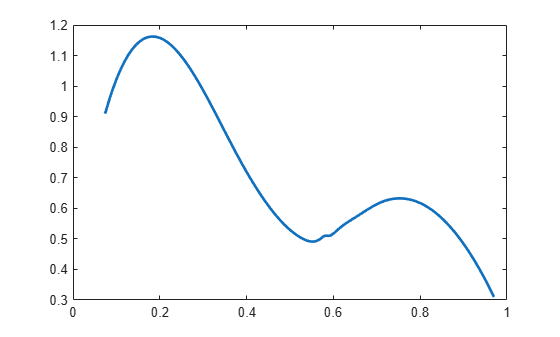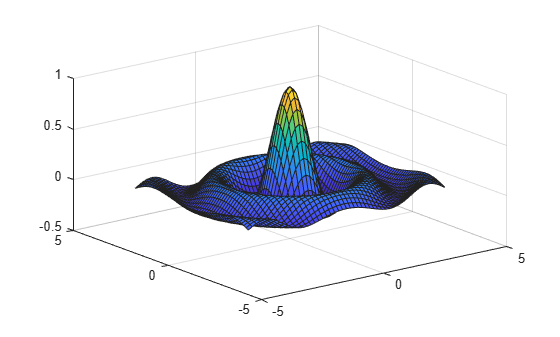Main Content

# fnval

Evaluate spline function

## Syntax

``v = fnval(f,x)``
``fnval(x,f) ``
``fnval(...,'l')``

## Description

example

````v = fnval(f,x)` provides the value f(x) at the points in `x` of the spline function f whose description is contained in `f`.If `f` is scalar-valued and univariate, the output `v` is obtained by replacing each entry of `x` by the value of f at that entry. This is the intent in all other cases, except that, for a `d`-valued `m`-variate function, `d`-vectors replaces `m`-vectors.For a univariate f : If f is scalar-valued, then v is of the same size as `x`. If f is `[d1,...,dr]`-valued, and `x` has size `[n1,...,ns]`, then `v` has size ```[d1,...,dr, n1,...,ns]```, with `v(:,...,:, j1,...,js)` the value of f at `x(j1,...,js)`, – except that: `n1` is ignored if it is `1` and `s` is `2`, i.e., if `x` is a row vector;MATLAB® ignores any trailing singleton dimensions of `x`. For an `m`-variate f with `m>1`, with f `[d1,...,dr]`-valued, `x` might be either an array, or else a cell array `{x1,...,xm}`.If `x` is an array, of size `[n1,...,ns]`, then `n1` must equal `m`, and `v` has size `[d1,...,dr, n2,...,ns]`, with ```v(:,...,:, j2,...,js)``` the value of f at `x(:,j2,...,js)`, – except that: `d1`, ..., `dr` is ignored in case f is scalar-valued, i.e., both `r` and `n1` are `1`;MATLAB ignores any trailing singleton dimensions of `x`. If `x` is a cell array, then it must be of the form `{x1,...,xm}`, with `xj` a vector, of length `nj`, and, in that case, `v` has size `[d1,...,dr, n1,...,nm]`, with `v(:,...,:, j1,...,jm)` the value of f at (`x1`(`j1`), ..., `xm`(`jm`)), – except that `d1`, ..., `dr` is ignored in case f is scalar-valued, i.e., both `r` and `n1` are `1`.If f has a jump discontinuity at `x`, then the value f(x +), i.e., the limit from the right, is returned, except when `x` equals the right end of the basic interval of the form; for such `x`, the value f(x–), i.e., the limit from the left, is returned.```
````fnval(x,f) ` is the same as `fnval(f,x)`.```
````fnval(...,'l')` treats f as continuous from the left. This means that if f has a jump discontinuity at `x`, then the value f(x–), i.e., the limit from the left, is returned, except when `x` equals the left end of the basic interval; for such `x`, the value f(x +) is returned.If the function is multivariate, then the above statements concerning continuity from the left and right apply coordinate wise.```

## Examples

collapse all

This example shows how to interpolate some data and plot and evaluate the resulting functions.

Define some data.

```x = [0.074 0.31 0.38 0.53 0.57 0.58 0.59 0.61 0.61 0.65 0.71 0.81 0.97]; y = [0.91 0.96 0.77 0.5 0.5 0.51 0.51 0.53 0.53 0.57 0.62 0.61 0.31]; ```

Interpolate the data and plot the resulting function, `f`.

`f = csapi( x, y )`
```f = struct with fields: form: 'pp' breaks: [1x12 double] coefs: [11x4 double] pieces: 11 order: 4 dim: 1 ```
`fnplt( f )`Find the value of the function `f` at `x = 0.5`.

`fnval( f, 0.5 )`
```ans = 0.5294 ```

Find the value of the function `f` at `0, 0.1, ..., 1`.

`fnval( f, 0:0.1:1 )`
```ans = 1×11 0.3652 1.0220 1.1579 0.9859 0.7192 0.5294 0.5171 0.6134 0.6172 0.4837 0.2156 ```

Create a function `f2` that represents a surface.

```x = 0.0001+(-4:0.2:4); y = -3:0.2:3; [yy, xx] = meshgrid( y, x ); r = pi*sqrt( xx.^2+yy.^2 ); z = sin( r )./r; f2 = csapi( {x,y}, z ); ```

Plot the function `f2`.

```fnplt( f2 ) axis( [-5, 5, -5, 5, -0.5, 1] );```Find the value of the function `f2` at `x = -2` and `y = 3`.

`fnval( f2, [-2; 3] )`
```ans = -0.0835 ```

## Input Arguments

collapse all

Spline function that you want to evaluate, specified as an object.

Points at which you want to evaluate the spline function `f`, specified as a vector, matrix or cell array.

## Output Arguments

collapse all

Value f(x) at the points in `x` of the spline function f, returned as a scalar, vector, matrix or cell array.

## Algorithms

For each entry of `x`, the function determines the relevant break-interval or knot-interval and assembles the relevant information. Depending on whether `f` is in ppform or in B-form, nested multiplication or the B-spline recurrence (see, e.g., [PGS; X.(3)]) are then used vector-fashion for the simultaneous evaluation at all entries of `x`. Evaluation of a multivariate polynomial spline function takes full advantage of the tensor product structure.

Evaluation of a rational spline follows up evaluation of the corresponding vector-valued spline by division of all but its last component by its last component.

Evaluation of a function in stform makes essential use of `stcol`, and tries to keep the matrices involved to reasonable size.

## See Also

### Topics

Introduced in R2006b

## Support

#### Machine Learning Challenges: Choosing the Best Classification Model and Avoiding Overfitting

Download white paper